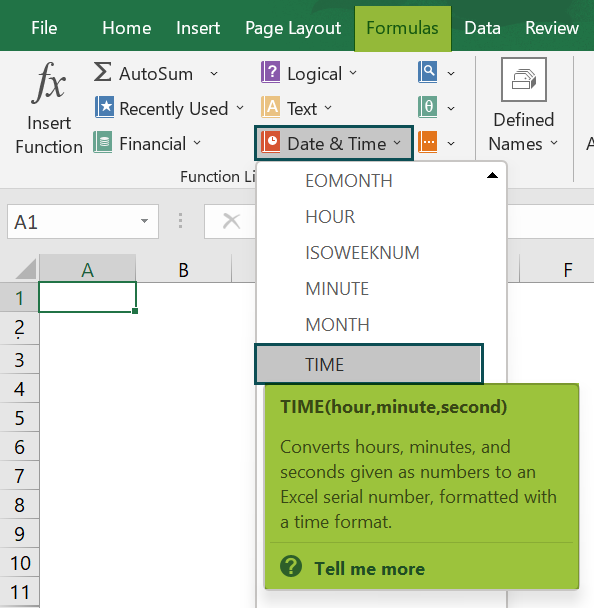# TIME Function In ExcelArticle byExcelMojo Team## What Is TIME Function In Excel?

The TIME Function in Excel calculates the required time and gives the output in the HHMM format. It is also calculated on the set parameters, i.e., if the Hours’ range is less than and equal to 12, then the time will be in AM, and post 12, the time will be in PM till 11:59 in the clock.

The TIME Excel Function is an inbuilt function which means we can insert the formula from the “Function Library” or enter it directly in the worksheet.

For example, we apply the TIME Function in Excel to return the time in the correct format.

The output is “12:20 PM”, as shown above.

###### Key Takeaways
• The TIME function in Excel takes numeric values as inputs, calculates the time, and returns the time as per the formula.
• If the cell format is General before the function is entered, the result will be in decimal.
• If the cell format is set as TIME before the function is entered, the result will be in time format.
• The TIME function returns a decimal value between 0 and 0.999988426, giving an hour, minute, and second value.
• The “#NUM!” error when the hour argument is negative, i.e., if the given hours are less than 0. It doesn’t occur for a negative value for a minute or a second.
• The “#VALUE!” error occurs when the arguments are non-numeric or alphanumeric.

### TIME() Excel Formula

The syntax of the TIME Excel formula is,

The arguments of the TIME Excel formula are,

• hour: It is the hour value of the time. If the value exceeds 23 hours, it gets divided by 24 hours, and the result becomes the hour value. It is a mandatory argument.
• minute: It is the minute value of the time. If the minute value exceeds 59, then 1 hour gets added to the hour value. It is a mandatory argument.
• second: It is the seconds’ value of the time. If the second’ value exceeds 59, then 1 minute gets added to the minute value. It is a mandatory argument.

### How to Use TIME Excel Function?

We can use the TIME function in Excel in 2 methods, namely,

1. Access from Ribbon in Excel.
2. Enter in the worksheet manually.

#### Method #1 – Access from the Excel ribbon

First, choose an empty cell for the output → select the “Formulas” tab → go to the “Functions Library” group → click the “Date & Time” drop-down → select the “TIME” function, as shown below.

The “Function Arguments” window appears. Enter the value in the “hour”, “minute”, and “second” arguments fields, and click “OK”, as shown below.

#### Method #2 – Enter in the worksheet manually

1. Select an empty cell for the output.
2. Type =TIME( in the selected cell. [Alternatively, type =T or =TI and double-click the TIME function from the Excel suggestions.]
3. Enter the arguments as values or cell references in Excel.
4. Press the “Enter” key.

We will calculate the time in the correct format using the TIME function in Excel example.

In the table, the data is,

• Column A contains the Hour.
• Column B contains the Minute.
• Column C contains the Second.
• Column D displays the Output.

The steps to calculate the time using the TIME function in Excel example are as follows:

1. Select cell D2 and enter the formula =TIME(A2, i.e., the hour value.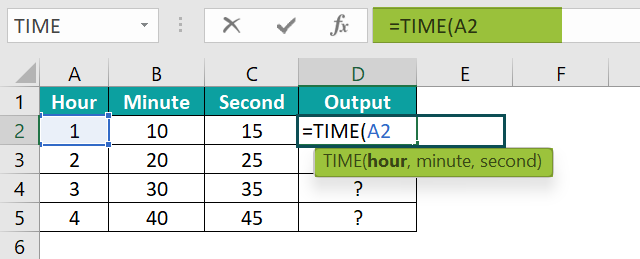2. Put a comma ‘,’, and enter the minute value, i.e., B2. The formula now is =TIME(A2,B2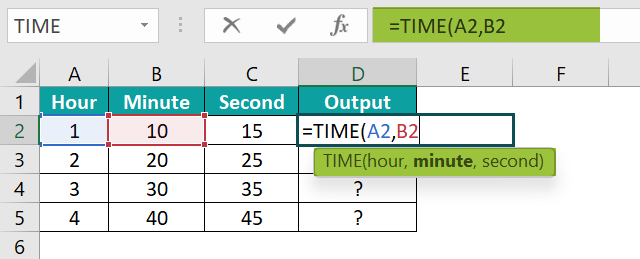3. Put a comma ‘,’, enter the seconds’ value, i.e., C2, and close the brackets. The complete formula is =TIME(A2,B2,C2)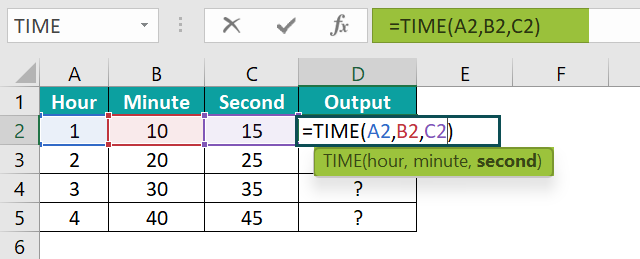4. Press the “Enter” key. The result in cell D2 is “1:10 AM”, as shown below.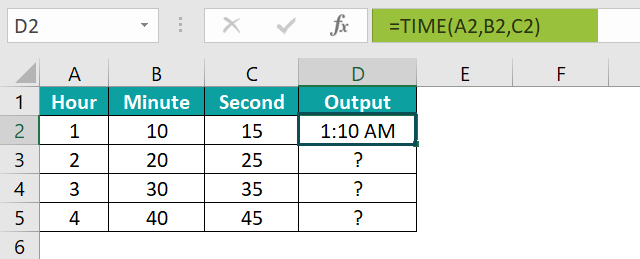5. Drag the formula from cell D2 to D5 using the fill handle.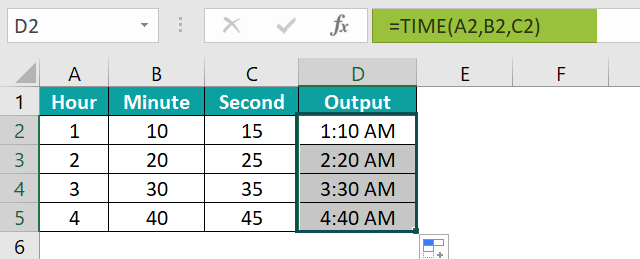The output is shown above.

### Examples

We will understand using TIME function in Excel with some advanced scenarios.

#### Example #1

We will calculate the time in the full-time format using TIME function in Excel.

In the table, the data is,

• Column A contains the Hour.
• Column B contains the Minute.
• Column C contains the Second.
• Column D displays the Output.

The steps to calculate the value using the TIME Function in Excel are as follows:

• Step 1: Select cell D2, enter the formula =TIME(A2,B2,C2), and press the “Enter” key. The result is “11:10:15 AM”, as shown below.
• Step 2: Drag the formula from cell D2 to D5 using the fill handle.

The output is shown above in the HHMMSS and the AM/PM format.

#### Example #2

We will calculate the time in the general and default time format using the TIME Function in Excel.

In the table, the data is,

• Column A contains the Hour.
• Column B contains the Minute.
• Column C contains the Second.
• Columns D and E display the Output.

The steps to calculate using the TIME Function in Excel are as follows:

• 1: Select cell D2, enter the formula =TIME(A2,B2,C2), and press the “Enter” key. The result is “0.906875”, as shown below.
• 2: Drag the formula from cell D2 to D5 using the fill handle. The output is shown below in General format.
• 3: To display the output in the default time format, select Column E → go to the “Home” tab → go to the “Numbers” group → click the “Number Format” drop-down → select the “Time” option as shown below.
• 4: Now, select cell E2, enter the formula again, i.e., =TIME(A2,B2,C2), and press the “Enter” key.
• 5: Drag the formula from cell E2 to E5 using the fill handle.

The output is shown above. Column E is now in default time format, and so are the values.

#### Example #3

We will calculate the time in the general, default, and full-time format using the TIME Function in Excel.

In the table, the data is,

• Column A contains the Hour.
• Column B contains the Minute.
• Column C contains the Second.
• Columns D, E, and F display the Output.

The steps to calculate the time using the TIME Function in Excel are as follows:

• 1: Select cell D2, enter the formula =TIME(A2,B2,C2), press the “Enter” key, and drag the formula from cell D2 to D5 using the fill handle, as shown below.
• 2: To display the output in the default time format, select Column E → go to the “Home” tab → go to the “Numbers” group à click the “Number Format” drop-down → select the “Time” option as shown below.
• 3: Now, select cell E2, enter the formula again, i.e., =TIME(A2,B2,C2), press the “Enter” key, and drag the formula from cell E2 to E5 using the fill handle, as shown below.
• 4: To display the output in the full-time format, select Column F → go to the “Home” tab → go to the “Numbers” group → click the “Number Format” drop-down → select the “More Number Formats…” option, as shown below.
• 5: In the “Format Cells” window that appears, select the “Number” tab → on the left, click the “Time” option from the “Category:” group → on the right, select the required format, i.e., the full-time format, from the “Type:” group → click “OK”, as shown below.
• 6: Now, select cell F2, enter the formula again, i.e., =TIME(A2,B2,C2), press the “Enter” key, and drag the formula from cell F2 to F5 using the fill handle, as shown below.

The output is shown above. Column D in the general time format, Column E in the default time format, and Column F in the full-time format.

### TIME Function Errors

• The TIME function in Excel calculates time as per the required format, i.e., General, default, or custom formats, for any given dataset.
• We get an error message when no arguments are provided in the formula
• The function allows us to create time with individual hour, minute, and second parameters, for a cell or a cell range.
• We can use the Time() function within different formulas and enter the current date, as the formula gets updated, the time also reflects accurately.

1. What is the TIME function used for in Excel?

The TIME function returns the time as a decimal number. We can format it to the HH:MM:SS format of time with AM or PM details. If the cell format is set as General before the function is entered, the result will be in decimal, but if the cell format is set as TIME before the function is entered, the result will be in time format.

The syntax of the TIME Function in Excel is =TIME(hour, minute, second)
For example, let us use the TIME function to return the time in the correct format.
First, the required time format is set in the cells.

Select cell A1, enter the formula =TIME(10,10,10), and press the “Enter” key. The output is “10:10 AM”.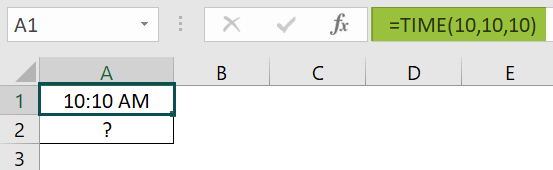Select cell A2, enter the formula =TIME(23,20,20), and press the “Enter” key. The output is “11:20 PM”.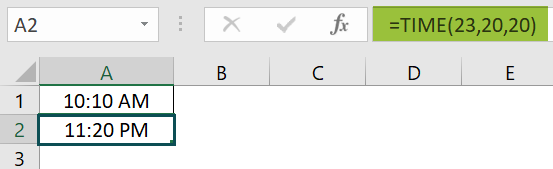The output is shown above.

2. How to insert the TIME function in Excel?

We can insert the TIME function in Excel as follows:
1.     Select an empty cell for the output.
2.     Type =TIME( in the selected cell. [Alternatively, type =T or =TI and double-click the TIME function from the Excel suggestions.]
3.     Enter the arguments as values or cell references.
4.     Press the “Enter” key.

3. Where is the TIME function in Excel?

The TIME Function in Excel is in the Formulas tab. We can insert the formula as follows:

First, choose an empty cell for the output → select the “Formulas” tab → go to the “Functions Library” group → click the “Date & Time” drop-down → select the “TIME” function, as shown below.